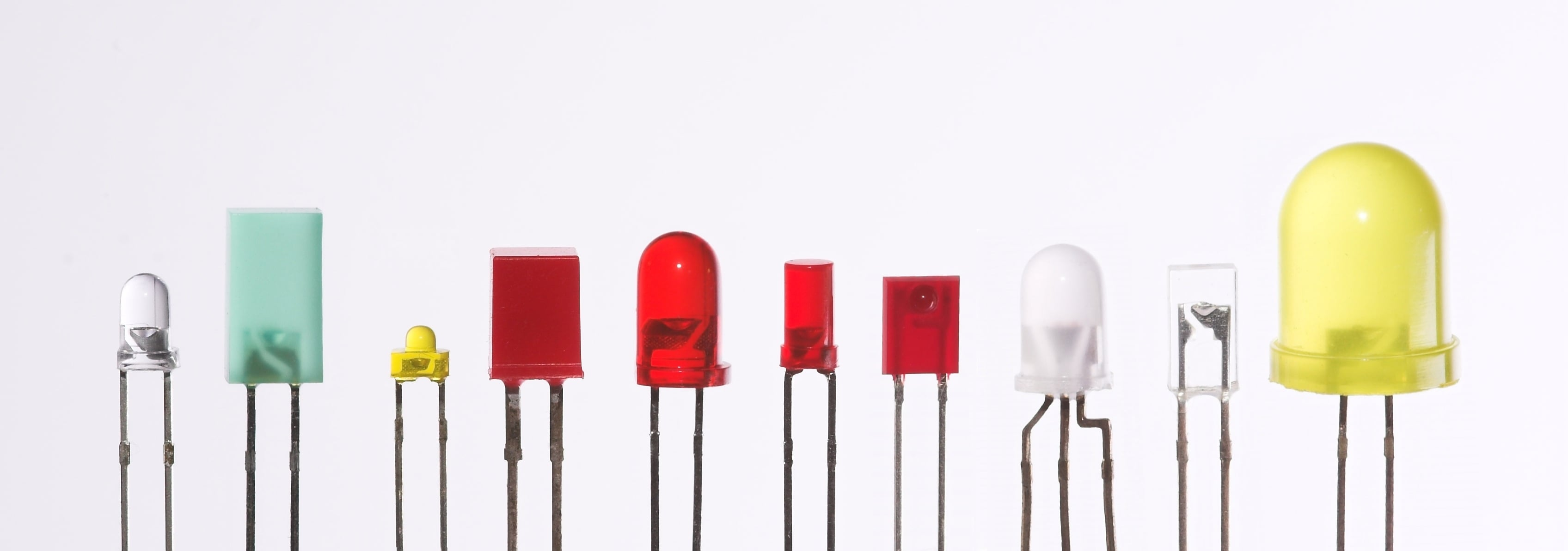# Convert MCD to Lumens

## This calculator is useful for calculating the luminous flux for LEDs where the angle is known.

### Inputs

Millicandelas (mcd)
Degrees

### Output

Lumens (lm)### Overview

Luminous flux (in lumens) is a measure of the total amount of light an LED puts out, while luminous intensity (in candelas) is a measure of how bright the beam in a particular direction is. This calculator gives the luminous flux emitted by an LED given its intensity and beam angle. LED datasheets often include the luminous intensity, so you might need to check them out. You will find this calculator useful if you want to compare the light output of LEDs with different beam angles.

### Equations

$$\Phi_{V} = I_{V} * \Omega$$

Where:

$$\Phi_{V}$$ = luminous flux in Lumens (lm)

$$I_{V}$$ = luminous intensity in Candela (cd)

$$\Omega$$ = solid angle of the beam in Steradian (sr)

Note that the calculator asks for a beam angle and not the solid angle. The formula used to convert beam angle to solid angle is:

$$\Omega = 2\pi(1-cos(\theta*\frac{\pi}{360}))$$

Where:

$$\theta$$ = beam angle in degrees

### Applications

It's a good practice to know the light intensity of the LED you will be using, especially if the LED is going to be used to illuminate a room. However, light intensity does not exactly tell how bright an LED is since it doesn't radiate light in all directions. Luminous flux is a better measurement because it considers the viewing angle.

Note that manufacturers often list the most favorable luminous intensity value for their particular component. In truth, there is no standard for measuring luminous intensity and so suppliers tend to give only the maximum value. This means that this calculator might provide results that are different from what is actually measured since it only takes the average luminous intensity across a given beam angle.